# Ex 2.2 NCERT Solutions - Polynomials Class 9 Notes | EduRev

## Class 9 : Ex 2.2 NCERT Solutions - Polynomials Class 9 Notes | EduRev

The document Ex 2.2 NCERT Solutions - Polynomials Class 9 Notes | EduRev is a part of the Class 9 Course Mathematics (Maths) Class 9.
All you need of Class 9 at this link: Class 9

ZEROES OF A POLYNOMIAL

Let p(x) is a polynomial. If p(a) = 0, then ‘a’ is said to be a zero of the polynomial p(x).

Note: (i) Finding the ‘zeros’ of a polynomial means solving the equation p(x) = 0.

(ii) A non-zero ‘constant polynomial’ has no zero.

(iii) Every real number is a zero of the zero polynomial.

(iv) Every linear polynomial has one and only one zero.

(v) A polynomial can have more than one zero.

(vi) A zero of a polynomial need not be 0.

(vii) ‘0’ may be a zero of a polynomial.

Ques 1. Find the value of the polynomial 5x – 4x2 + 3 at (i) x = 0 (ii) x = –1 (iii) x = 2

Ans: (i) ∵ p(x) = 5x – 4x2 + 3 = 5(x) – 4(x)2 + 3

∴ p(0) = 5(0) – 4(0) + 3 = 0 – 0 + 3 = 3

Thus, the value of 5x – 4x2 + 3 at x = 0 is 3.

(ii) ∵ p(x) = 5x – 4x2 + 3 = 5(x) – 4(x)2 + 3

∴ p(–1) = 5(–1) – 4(–1)+ 3 = –5 – 4(1) + 3 = –5 – 4 + 3 = –9 + 3 = –6

∴ The value of 5x – 4x2 + 3 at x = –1 is –6.

(iii) ∵ p(x) = 5x – 4x2 + 3 = 5(x) – 4(x)2 + 3

∴ p(2) = 5(2) – 4(2)2 + 3 = 10 – 4(4) + 3 = 10 – 16 + 3 = –3

Thus the value of 5x – 4x2 + 3 at x = 2 is –3.

Ques 2. Find p(0), p(1) and p(2) for each of the following polynomials: (i) p(y) = y2 – y + 1 (ii) p(t) = 2 + t + 2t2 – t3 (iii) p(x) = x3 (iv) p(x) = (x – 1) (x + 1)

Ans: (i) p(y) = y2 – y + 1

∵ p(y) = y2 – y + 1 = (y)– y + 1

∴ p(0) = (0)2 – (0) + 1 = 0 – 0 + 1 = 1

p(1) = (1)– (1) + 1 = 1 – 1 + 1 = 1

p(2) = (2)2 –2 + 1 = 4 – 2 + 1 = 3

(ii) p(t) = 2 + t + 2t2 – t3

∵ p(t) = 2 + t + 2t2 – t3 = 2 + t + 2(t)2 – (t)3

∴ p(0) = 2 + (0) + 2(0)2 – (0)3 = 2 + 0 + 0 – 0 = 2

p(1) = 2 + (1) + 2 (1)– (1)= 2 + 1 + 2 – 1 = 4

p(2) = 2 + 2 + 2(2)2 – (2)3 = 2 + 2 + 8 – 8 = 4

(iii) p(x) = x3 ∵ p(x) = x= (x)

∴ p(0) = (0)= 0 p(1) = (1)3 = 1

p(2) = (2)3 = 8 [∵ 2 x2 x2 = 8]

(iv) p(x) = (x – 1)(x + 1)

∵ p(x) = (x – 1)(x + 1)

∴ p(0) = (0 – 1)(0 + 1) = –1 x 1 = –1

p(1) = (1 – 1)(1 + 1) = (0)(2) = 0

p(2) = (2 – 1)(2 + 1) = (1)(3) = 3

Ques 3. Verify whether the following are zeros of the polynomial, indicated against them.

(i) p(x) = 3x + 1, x =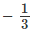(ii) p(x) = 5x – p , x =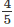(iii) p(x) = x2 – 1, x = 1, – 1
(iv) p(x) = (x + 1) (x – 2), x = –1, 2
(v) p(x) = x2, x = 0
(vi) p(x) = lx + m, x =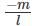(vii) p(x) = 3x2 – 1, x =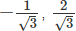(viii) p(x) = 2x + 1, x =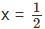Ans: (i) ∵ p(x) = 3x + 1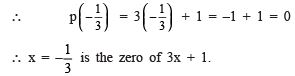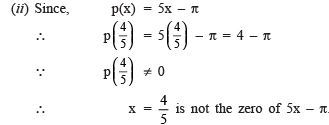(iii) Since, p(x) = x2 – 1

∴ p(1) = (1)2 – 1 = 1 – 1 = 0

Since, p(1) = 0,

∴ x = 1 is a zero of x2 – 1.

Also p(–1) = (–1)– 1 = 1 – 1 = 0 i.e. p(–1) = 0,

∴ x = –1 is also a zero of x2 – 1.

(iv) We have p(x) = (x + 1)(x – 2)

∴ p(–1) = (–1 + 1)(–1 – 2) = (0)(–3) = 0

Since p(–1) = 0,

∴ x = –1 is a zero of (x + 1) (x – 1).

Also, p(2) = (2 + 1)(2 – 2) = (3)(0) = 0

Since p(2) = 0,

∴ x = 2 is also a zero of (x + 1)(x – 1).

(v) We have p(x) = x

∴ p(0) = (0)2 = 0 Since p(0) = 0,

∴ 0 is a zero of x2.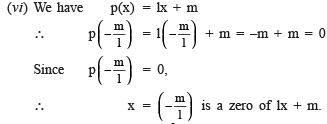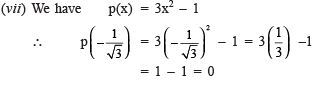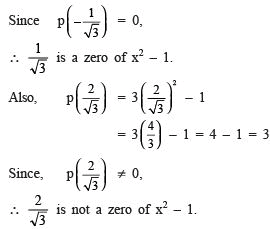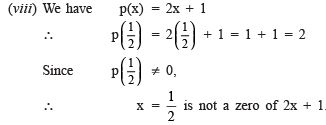Ques 4. Find the zero of the polynomial in each of the following cases: (i) p(x) = x + 5 (ii) p(x) = x – 5 (iii) p(x) = 2x + 5 (iv) p(x) = 3x – 2 ( v ) p(x) = 3x (vi) p(x) = ax, a ≠ 0 (vii) p(x) = cx + d, c ≠ 0, c, d are real numbers.
Ans:
(i) We have p(x) = x + 5

∴ p(x) = 0

⇒ x + 5 = 0 or x = –5

Thus, a zero of x + 5 is (–5).

(ii) We have p(x) = x – 5

∴ p(x) = 0

⇒ x – 5 = 0 or x = 5

Thus, a zero of x – 5 is 5.

(iii) We have p(x) = 2x + 5

∴ p(x) = 0

⇒ 2x + 5 = 0 or 2x = –5 or x =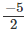Thus, a zero of 2x + 5 is(iv) Since p(x) = 3x – 2 ∴ p(x) = 0

⇒ 3x – 2 = 0 or 3x = 2 or x = 2/3

Thus, a zero of 3x – 2 is 2/3

(v) Since p(x) = 3x ∴ p(x) = 0

⇒ 3x = 0 or x = 0/3 or 0

Thus, a zero of 3x is 0.

(vi) Since, p(x) = ax, a 0

⇒ p(x) = 0

⇒ ax = 0 or x = 0 a  = 0

Thus, a zero of ax is 0.

(vii) Since, p(x) = cx + d

∴ p(x) = 0

⇒ cx + d = 0 or c x = –d or x = – dc

Thus, a zero of cx + d is – dc.

Ques 5: If p(x) = x2 – 4x + 3, evaluate: p(2) – p(–1) +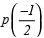Ans: We have p(x) = x2 – 4x + 3

∴ p(–1) = (–1)2 – 4(–1) + 3

= 1 + 4 + 3 = 8

and p(2) = (2)2 – 4(2) + 3 = 4 – 8 + 3 = –1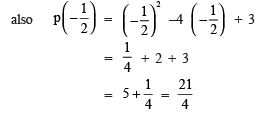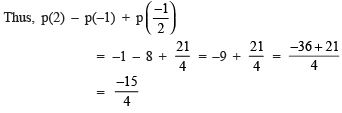Offer running on EduRev: Apply code STAYHOME200 to get INR 200 off on our premium plan EduRev Infinity!

## Mathematics (Maths) Class 9

190 videos|233 docs|82 tests

,

,

,

,

,

,

,

,

,

,

,

,

,

,

,

,

,

,

,

,

,

;